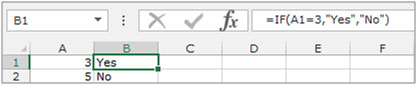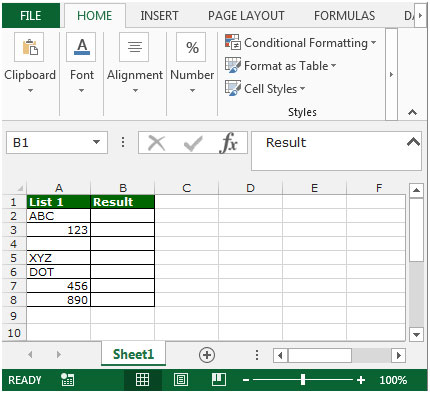Creating Formulas that Only Return Results from Non-Blank Cells in Microsoft Excel 2010

To create formulas that only return results from non-blank cells we can use the “ISBLANK” and “IF”formulasin Microsoft Excel 2010.

ISBLANK:This function can be used tocheck for blank or null values in a range.

Syntax of “ISBLANK” function:    =ISBLANK (value)

Example:Cell A2 contains the textXYZ

=ISBLANK (A2), function will return“FALSE”

Cell A3contains the textXYZ

=ISBLANK (A3), function will return “TRUE”

IF:- IF condition allows us to use multiple conditions in a cell.  It helps us to make the function flexible and Excel allows us to use a maximum of 64 conditions in a cell.

Syntax of “IF” function:    =if (logical test, [value_if_true], [value_if_false])

Example:Cells A2 and A3 contain the numbers 3 and 5. If the number is 3 the formula should display “Yes” otherwise “No”.

=IF (A1=3,”Yes”,”No”)Lets take an example to understand.  We have data in range A2:A7, in which some cells are blanks, and few cells contains text and some cells contain numbers.To create a formula that only returns a result from the non-blank cells, do the following –

• Select the cell B2
• Enter the formula =IF(ISBLANK(A2)=FALSE,A2,””)
• Press enter on the keyboard.
• The function will return the same text which is cell A2 contains.
• To return the value for all rest of cells, copy the same formula and paste in the range B3:B8.
• This way we can count the non-blank cells in the range.Another method to return results for non-blank cells is to simply use the IF function.
Instead of =IF(ISBLANK(A2)=FALSE, A2, “”), you can use =IF(A2=””,””,A2) or =IF(A2<>””,A2,””).  The first option =IF(A2=””,””,A2) checks if A2 is blank.  If it is blank, a blank cell is returned.  If its not blank, the contents of A2 are returned.

Similarly, in the case of =IF(A2<>””,A2,””) check if A2 is not blank.  If its true, A2 is returned, else a blank cell is returned.  “<>” is used to denote “not equal to”.

Depending on the option you choose, you can then copy the formula to the rest of the column.

This is how we can create the formulas that only return results from non blank cells in Microsoft Excel.

## Users are saying about us...

1.I have a date in Column A, and I have a formula in Column H as follows: =SUM(A3+30)
So a date appears in Column H which is 30 days after the date in Column A. Problem is that if there is no date in Column A, this appears in Column H: #VALUE! Is there any way to prevent this? I’ve spent days trying to figure this out. Greatly appreciated!!!!

•Try this formula in H3, assuming A3 is the source date.
=IF(ISBLANK(A3)=FALSE,(A3+30),””)

•The following formula is not working for me =IF(ISBLANK(B4)=FALSE,DAY(B4),””)

Here B4 contain a Date. The out put is #NAME?

My requirement is needs a blank cell instead of 0 in other cell if the B4 cell is blank.

•Thanks, Dan! worked perfectly!!!

•Thanks Dan!

2.Dan Akers’ formula was a big help to me. I have a running list and wanted to auto-number each line as new items were added (ie, when something is typed into a field on row 7, another cell on that row would auto-enter a “7″ to keep track of how many lines are populated). It’s a stupid calculation but I didn’t want to drag out 1000 numbers for empty rows. =IF(ISBLANK(B82)=FALSE,(A81+1),””) worked like a champ. Thanks again.

3.The following formula is not working for me =IF(ISBLANK(B4)=FALSE,DAY(B4),””)

Here B4 contain a Date. The out put is #NAME?

My requirement is needs a blank cell instead of 0 in other cell if the B4 cell is blank.

4.I have a spreadsheet where a due date in column H and date mailed in Column I. i have a calculation in column J to see if we met the 15 day requirement yes or no: =IF(AND(I2<=H2),"Yes","No").
Problem is its displaying yes in all the fields that don't even have a due date yet. How can I get this not to show anything if a there is no due date entered?

Terms and Conditions of use

The applications/code on this site are distributed as is and without warranties or liability. In no event shall the owner of the copyrights, or the authors of the applications/code be liable for any loss of profit, any problems or any damage resulting from the use or evaluation of the applications/code.